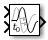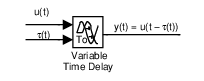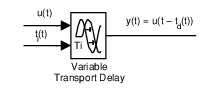Variable Time Delay

Delay input by variable amount of time

• Library:

•Description

The Variable Transport Delay and Variable Time Delay blocks appear as two blocks in the Simulink® block library. However, they are the same Simulink block with different settings for the Select delay type parameter. Use this parameter to specify the mode in which the block operates.

Variable Time Delay

In this mode, the block has a data input, a time delay input, and a data output. (See Port Location After Rotating or Flipping for a description of the port order for various block orientations.) The output at the current time step equals the value of its data input at a previous time step. This time step is the current simulation time minus a delay time specified by the time delay input.

$y\left(t\right)=u\left(t-{t}_{0}\right)=u\left(t-\tau \left(t\right)\right)$During the simulation, the block stores time and input value pairs in an internal buffer. At the start of simulation, the block outputs the value of the Initial output parameter until the simulation time exceeds the time delay input. Then, at each simulation step, the block outputs the signal at the time that corresponds to the current simulation time minus the delay time.

If you want the output at a time between input storing times and the solver is a continuous solver, the block interpolates linearly between points. If the time delay is smaller than the step size, the block extrapolates an output point from a previous point. For example, consider a fixed-step simulation with a step size of 1 and the current time at t = 5. If the delay is 0.5, the block must generate a point at t = 4.5, but the most recent stored time value is at t = 4. Thus, the block extrapolates the input at 4.5 from the input at 4 and uses the extrapolated value as its output at t = 5.

Extrapolating forward from the previous time step can produce a less accurate result than extrapolating back from the current time step. However, the block cannot use the current input to calculate its output value because the input port does not have direct feedthrough.

If the model specifies a discrete solver, the block does not interpolate between time steps. Instead, it returns the nearest stored value that precedes the required value.

Variable Transport Delay

In this mode, the block output at the current time step is equal to the value of its data (top, or left) input at an earlier time step equal to the current time minus a transport delay.

$y\left(t\right)=u\left(t-{t}_{d}\left(t\right)\right)$

Simulink software finds the transport delay, ${t}_{d}\left(t\right)$, by solving the following equation:

${\int }_{t-{t}_{d}\left(t\right)}^{t}\frac{1}{{t}_{i}\left(\tau \right)}d\tau =1$

This equation involves an instantaneous time delay, ${t}_{i}\left(t\right)$, given by the time delay (bottom, or right) input.Suppose that you want to use this block to model the fluid flow through a pipe where the fluid speed varies with time. In this case, the time delay input to the block is

${t}_{i}\left(t\right)=\frac{L}{{v}_{i}\left(t\right)}$

where L is the length of the pipe and ${v}_{i}\left(t\right)$ is the speed of the fluid.

Ports

Input

expand all

Input signal specified as a scalar, vector, or matrix.

Data Types: double

Time delay input specified as a scalar, vector, or matrix. When the block is in Variable time delay mode, this value specifies the time delay. For more information about that calculation, see Variable Time Delay.

Dependencies

To enable this port, set Select delay type to Variable time delay.

Data Types: double

Instantaneous time delay input specified as a scalar, vector, or matrix. When the block is in Variable transport delay mode, this value is used to calculate the transport delay. For more information about that calculation, see Variable Transport Delay.

Dependencies

To enable this port, set Select delay type to Variable transport delay.

Data Types: double

Output

expand all

Output signal specified as a scalar, vector, or matrix.

Data Types: double

Parameters

expand all

Specify the type of delay as Variable time delay or Variable transport delay.

The default value of this parameter depends on the block implementation: Variable time delay for the Variable Time Delay block, and Variable transport delay for the Variable Transport Delay block.

Dependencies

• Setting this parameter to Variable time delay enables the Handle zero delay parameter.

• Setting this parameter to Variable transport delay enables the Absolute tolerance and State Name parameters.

Programmatic Use

 Block Parameter: VariableDelayType Type: character vector, string Values: 'Variable transport delay' | 'Variable time delay'

Set the maximum value of the time delay input. This value defines the largest time delay input that this block allows. The block clips any delay that exceeds this value. This value cannot be negative. If the time delay becomes negative, the block clips it to zero and issues a warning message.

Programmatic Use

 Block Parameter: MaximumDelay Type: character vector, string Value: scalar | vector Default: '10'

Specify the output that the block generates until the simulation time first exceeds the time delay input.

Dependencies

• The initial output of this block cannot be inf or NaN.

• A Run-to-run tunable parameter cannot be changed during simulation run time. However, changing it before a simulation begins does not cause Accelerator or Rapid Accelerator to regenerate code.

Programmatic Use

 Block Parameter: InitialOutput Type: character vector, string Values: scalar | vector Default: '0'

Define the initial memory allocation for the number of input points to store. The input points define the history of the input signal up to the current simulation time.

• If the number of input points exceeds the initial buffer size, the block allocates additional memory.

• After simulation ends, a message displays if the buffer is not sufficient and more memory must be allocated.

Tips

• Because allocating memory slows down simulation, choose this value carefully if simulation speed is an issue.

• For long time delays, this block might use a large amount of memory, particularly for dimensionalized input.

Programmatic Use

 Block Parameter: MaximumPoints Type: character vector, string Values: scalar | vector Default: '1024'

Selecting this check box uses a fixed-size buffer to save input data from previous time steps. When you clear this check box, the block does not use a fixed-size buffer.

The Initial buffer size parameter specifies the buffer size. If the buffer is full, new data replaces data already in the buffer. Simulink software uses linear extrapolation to estimate output values that are not in the buffer.

Note

ERT or GRT code generation uses a fixed-size buffer even if you do not select this check box.

Tips

• If the input data is linear, selecting this check box can save memory.

• If the input data is nonlinear, do not select this check box. Doing so might yield inaccurate results.

Programmatic Use

 Block Parameter: FixedBuffer Type: character vector, string Values: 'off' | 'on' Default: 'off'

Selecting this check box converts this block to a direct feedthrough block. When you clear this check box, the block does not use direct feedthrough.

Dependencies

To enable this parameter, set Select delay type to Variable time delay.

Programmatic Use

 Block Parameter: ZeroDelay Type: character vector, string Values: 'off' | 'on' Default: 'off'

When you select this parameter, the block outputs its input during linearization and trim, which sets the block mode to direct feedthrough. To disable direct feedthrough, clear this check box.

Tips

• Selecting this check box can cause a change in the ordering of states in the model when you use the functions linmod, dlinmod, or trim. To extract this new state ordering:

1. Compile the model using the following command, where model is the name of the Simulink model.

[sizes, x0, x_str] = model([],[],[],'lincompile');
2. Terminate the compilation with the following command.

model([],[],[],'term');
• The output argument x_str, which is a cell array of the states in the Simulink model, contains the new state ordering. When you pass a vector of states as input to the linmod, dlinmod, or trim functions, the state vector must use this new state ordering.

Programmatic Use

 Block Parameter: TransDelayFeedthrough Type: character vector, string Values: 'off' | 'on' Default: 'off'

Set the order of the Pade approximation for linearization routines.

• The default value is 0, which results in a unity gain with no dynamic states.

• Setting the order to a positive integer n adds n states to your model, but results in a more accurate linear model of the transport delay.

Programmatic Use

 Block Parameter: PadeOrder Type: character vector, string Values: scalar Default: '0'

Specify the absolute tolerance for computing the block state.

Dependencies

To enable this parameter, set Select delay type to Variable transport delay.

Programmatic Use

 Block Parameter: AbsoluteTolerance Type: character vector, string Values: 'auto' | '-1' | any positive real scalar or vector Default: 'auto'

Assign a unique name to each state. If this field is blank, no name assignment occurs.

Tips

• To assign a name to a single state, enter the name between quotes, for example, 'velocity'.

• To assign names to multiple states, enter a comma-delimited list surrounded by braces, for example, {'a', 'b', 'c'}. Each name must be unique.

• The state names apply only to the selected block.

• The number of states must divide evenly among the number of state names.

• You can specify fewer names than states, but you cannot specify more names than states.

For example, you can specify two names in a system with four states. The first name applies to the first two states and the second name to the last two states.

• To assign state names with a variable in the MATLAB® workspace, enter the variable without quotes. A variable can be a character vector, string, cell array, or structure.

Dependencies

To enable this parameter, set Select delay type to Variable transport delay.

Programmatic Use

 Block Parameter: ContinuousStateAttributes Type: character vector, string Values: ' ' | user-defined character vector, user-defined string Default: ' '

Block Characteristics

 Data Types double Direct Feedthrough yes Multidimensional Signals no Variable-Size Signals no Zero-Crossing Detection no